Next: 7.2 Convergence Up: 7. Complex Sequences Previous: 7. Complex Sequences   Index

# 7.1 Some Examples.

In definition 5.1 , we defined a sequence in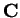to be a function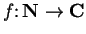. Since we are identifyingwith a subset of, every sequence inis also a sequence in, and all of our results for complex sequences are applicable to real sequences.

7.1   Notation (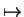) I will say  consider the sequence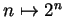" or  consider the sequence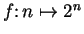" to mean  consider the sequencesuch that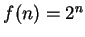for all". The arrowis read  maps to".

7.2   Definition (Geometric sequence.) For each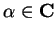, the sequence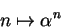is called the geometric sequence with ratio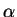.

I will often represent a sequence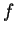inby a polygonal line with vertices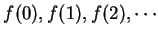. The two figures below represent geometric sequences with ratios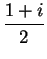and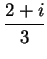respectively.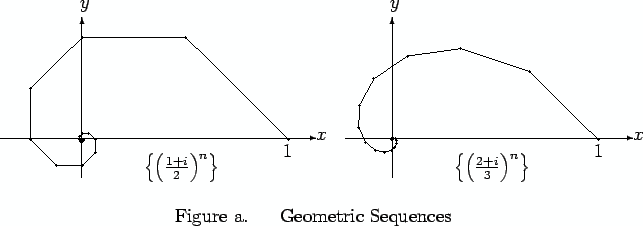7.3   Definition (Geometric series.) If, then the sequenceis called the geometric series with ratio.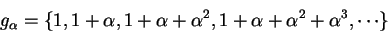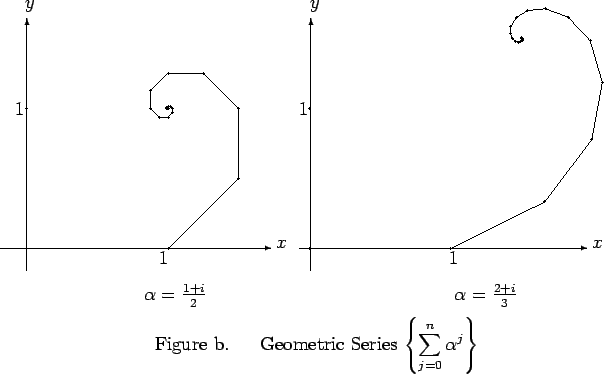Figure b shows the geometric series corresponding to the geometric sequences in figure a. If you examine the figures you should notice a remarkable similarity between the figure representingand the figure representing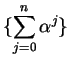.

7.4   Entertainment. Describe the apparent similarity between the figure forand the figure for. Then prove that this similarity is really present for all.

7.5   Definition (Constant sequence.) For each, let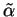, denote the constant sequence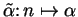; i.e.,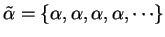.Next: 7.2 Convergence Up: 7. Complex Sequences Previous: 7. Complex Sequences   Index